# Top 40 Java 8 Interview Questions & Answers [Most Important]

In this Tutorial, we have provided the Most Important Java 8 Interview Questions & their Answers with Code Examples & Explanation:

All the important questions that are listed in this tutorial are specific to Java 8. Java has evolved a lot (over time) with the introduction of new versions. With each version, we have new features associated with Java. All these important features will be covered in this tutorial.

These are very common questions which you will be asked in any Java interviews that demand advanced skills. These concepts are must-have if you are going to appear for any standard Java Certification Exams like Oracle Certified Associate (OCA).

This article will be very much suitable for both Java Developers as well as Java Testers/Automation Testers or anyone who is looking for higher pay in the same field because it demands advanced Java skills.

### Most Frequently Asked Java 8 Interview Questions

Q #1) List down the new features introduced in Java 8?

Answer: New features that are introduced in Java 8 are enlisted below:

• Lambda Expressions
• Method References
• Optional Class
• Functional Interface
• Default methods
• Nashorn, JavaScript Engine
• Stream API
• Date API

Q #2) What are Functional Interfaces?

Answer: Functional Interface is an interface that has only one abstract method. The implementation of these interfaces is provided using a Lambda Expression which means that to use the Lambda Expression, you need to create a new functional interface or you can use the predefined functional interface of Java 8.

The annotation used for creating a new Functional Interface is “@FunctionalInterface”.

Q #3) What is an optional class?

Answer: Optional class is a special wrapper class introduced in Java 8 which is used to avoid NullPointerExceptions. This final class is present under java.util package. NullPointerExceptions occurs when we fail to perform the Null checks.

Q #4) What are the default methods?

Answer: Default methods are the methods of the Interface which has a body. These methods, as the name suggests, use the default keywords. The use of these default methods is “Backward Compatibility” which means if JDK modifies any Interface (without default method) then the classes which implement this Interface will break.

On the other hand, if you add the default method in an Interface then you will be able to provide the default implementation. This won’t affect the implementing classes.

Syntax:

 `public` `interface` `questions{``        ``default` `void` `print() {``System.out.println(``"https://softwaredaily01.com/"``);``             ``}``    ``}`

Q #5) What are the main characteristics of the Lambda Function?

Answer: Main characteristics of the Lambda Function are as follows:

• A method that is defined as Lambda Expression can be passed as a parameter to another method.
• A method can exist standalone without belonging to a class.
• There is no need to declare the parameter type because the compiler can fetch the type from the parameter’s value.
• We can use parentheses when using multiple parameters but there is no need to have parenthesis when we use a single parameter.
• If the body of expression has a single statement then there is no need to include curly braces.

Q #6) What was wrong with the old date and time?

Answer: Enlisted below are the drawbacks of the old date and time:

• Java.util.Date is mutable and is not thread-safe whereas the new Java 8 Date and Time API are thread-safe.
• Java 8 Date and Time API meets the ISO standards whereas the old date and time were poorly designed.
• It has introduced several API classes for a date like LocalDate, LocalTime, LocalDateTime, etc.
• Talking about the performance between the two, Java 8 works faster than the old regime of date and time.

Q #7) What is the difference between the Collection API and Stream API?

Answer: The difference between the Stream API and the Collection API can be understood from the below table:

Stream APICollection API
It was introduced in Java 8 Standard Edition version.It was introduced in Java version 1.2
There is no use of the Iterator and Spliterators.With the help of forEach, we can use the Iterator and Spliterators to iterate the elements and perform an action on each item or the element.
An infinite number of features can be stored.A countable number of elements can be stored.
Consumption and Iteration of elements from the Stream object can be done only once.Consumption and Iteration of elements from the Collection object can be done multiple times.
It is used to compute data.It is used to store data.

Q #8) How can you create a Functional Interface?

Answer: Although Java can identify a Functional Interface, you can define one with the annotation

@FunctionalInterface

Once you have defined the functional interface, you can have only one abstract method. Since you have only one abstract method, you can write multiple static methods and default methods.

Below is the programming example of FunctionalInterface written for multiplication of two numbers.

 `@FunctionalInterface` `// annotation for functional interface``interface` `FuncInterface {``    ``public` `int` `multiply(``int` `a, ``int` `b);``}``public` `class` `Java8 {``   ``public` `static` `void` `main(String args[]) {``        ``FuncInterface Total = (a, b) -> a * b;``        ``// simple operation of multiplication of 'a' and 'b'``        ``System.out.println(``"Result: "``+Total.multiply(``30``, ``60``));``    ``}``}`

Output: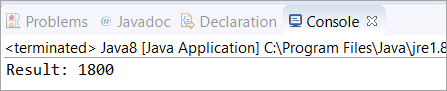Q #9) What is a SAM Interface?

Answer: Java 8 has introduced the concept of FunctionalInterface that can have only one abstract method. Since these Interfaces specify only one abstract method, they are sometimes called as SAM Interfaces. SAM stands for “Single Abstract Method”.

Q #10) What is Method Reference?

Answer: In Java 8, a new feature was introduced known as Method Reference. This is used to refer to the method of functional interface. It can be used to replace Lambda Expression while referring to a method.

For Example: If the Lambda Expression looks like

`num -> System.out.println(num)`

Then the corresponding Method Reference would be,

`System.out::println`

where “::” is an operator that distinguishes class name from the method name.

Q #11) Explain the following Syntax

`String:: Valueof Expression`

Answer: It is a static method reference to the ValueOf method of the String class. System.out::println is a static method reference to println method of out object of System class.

It returns the corresponding string representation of the argument that is passed. The argument can be Character, Integer, Boolean, and so on.

Q #12) What is a Predicate? State the difference between a Predicate and a Function?

Answer: Predicate is a pre-defined Functional Interface. It is under java.util.function.Predicate package. It accepts only a single argument which is in the form as shown below,

Predicate<T>

PredicateFunction
It has the return type as Boolean.It has the return type as Object.
It is written in the form of Predicate< T> which accepts a single argument.It is written in the form of Function< T, R> which also accepts a single argument.
It is a Functional Interface which is used to evaluate Lambda Expressions. This can be used as a target for a Method Reference.It is also a Functional Interface which is used to evaluate Lambda Expressions. In Function< T, R>, T is for input type and R is for the result type. This can also be used as a target for a Lambda Expression and Method Reference.

Q #13) Is there anything wrong with the following code? Will it compile or give any specific error?

 `@FunctionalInterface``public` `interface` `Test {``    ``public` `C apply(A a, B b);`` ``    ``default` `void` `printString() {``        ``System.out.println(``"softwaretestinghelp"``);``    ``}``}`

Answer: Yes. The code will compile because it follows the functional interface specification of defining only a single abstract method. The second method, printString(), is a default method that does not count as an abstract method.

Q #14) What is a Stream API? Why do we require the Stream API?

Answer: Stream API is a new feature added in Java 8. It is a special class that is used for processing objects from a source such as Collection.

We require the Stream API because,

• It supports aggregate operations which makes the processing simple.
• It supports Functional-Style programming.
• It does faster processing. Hence, it is apt for better performance.
• It allows parallel operations.

Q #15) What is the difference between limit and skip?

Answer: The limit() method is used to return the Stream of the specified size. For Example, If you have mentioned limit(5), then the number of output elements would be 5.

Let’s consider the following example. The output here returns six elements as the limit is set to ‘six’.

 `import` `java.util.stream.Stream;``public` `class` `Java8 {``    ``    ``public` `static` `void` `main(String[] args) {``        ``Stream.of(``0``,``1``,``2``,``3``,``4``,``5``,``6``,``7``,``8``)``        ``.limit(``6``)         ``        ``/*limit is set to 6, hence it will print the ``        ``numbers starting from 0 to 5 ``        ``*/``        ``.forEach(num->System.out.print(``"\n"``+num));``    ``}``}`

Output: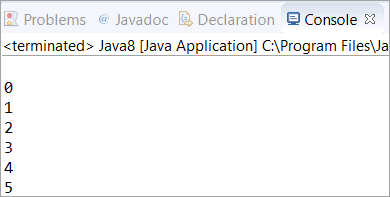Whereas, the skip() method is used to skip the element.

Let’s consider the following example. In the output, the elements are 6, 7, 8 which means it has skipped the elements till the 6th index (starting from 1).

 `import` `java.util.stream.Stream;``public` `class` `Java8 {``    ``    ``public` `static` `void` `main(String[] args) {``        ``Stream.of(``0``,``1``,``2``,``3``,``4``,``5``,``6``,``7``,``8``)``        ``.skip(``6``)``        ``/*``         ``It will skip till 6th index. Hence 7th, 8th and 9th``         ``index elements will be printed``         ``*/``        ``.forEach(num->System.out.print(``"\n"``+num));``    ``}``}`

Output: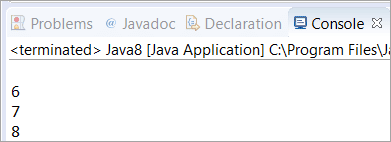Q #16) How will you get the current date and time using Java 8 Date and Time API?

Answer: The below program is written with the help of the new API introduced in Java 8. We have made use of LocalDate, LocalTime, and LocalDateTime API to get the current date and time.

In the first and second print statement, we have retrieved the current date and time from the system clock with the time-zone set as default. In the third print statement, we have used LocalDateTime API which will print both date and time.

 `class` `Java8 {``    ``public` `static` `void` `main(String[] args) {``        ``System.out.println(``"Current Local Date: "` `+ java.time.LocalDate.now());``        ``//Used LocalDate API to get the date``        ``System.out.println(``"Current Local Time: "` `+ java.time.LocalTime.now());``        ``//Used LocalTime API to get the time``        ``System.out.println(``"Current Local Date and Time: "` `+ java.time.LocalDateTime.now());``        ``//Used LocalDateTime API to get both date and time``    ``}``}`

Output: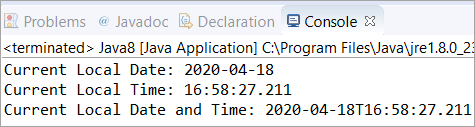Q #17) What is the purpose of the limit() method in Java 8?

Answer: The Stream.limit() method specifies the limit of the elements. The size that you specify in the limit(X), it will return the Stream of the size of ‘X’. It is a method of java.util.stream.Stream

Syntax:

`limit(X)`

Where ‘X’ is the size of the element.

Q #18) Write a program to print 5 random numbers using forEach in Java 8?

Answer: The below program generates 5 random numbers with the help of forEach in Java 8. You can set the limit variable to any number depending on how many random numbers you want to generate.

 `import` `java.util.Random;``class` `Java8 {``    ``public` `static` `void` `main(String[] args) {``        ``Random random = ``new` `Random();``        ``random.ints().limit(``5``).forEach(System.out::println);``        ``/* limit is set to 5 which means only 5 numbers will be printed``        ``with the help of terminal operation forEach``        ``*/``    ``}``}`

Output: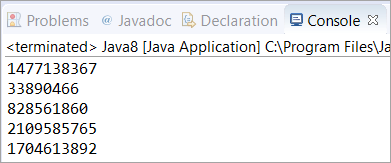Q #19) Write a program to print 5 random numbers in sorted order using forEach in Java 8?

Answer: The below program generates 5 random numbers with the help of forEach in Java 8. You can set the limit variable to any number depending on how many random numbers you want to generate. The only thing you need to add here is the sorted() method.

 `import` `java.util.Random;``class` `Java8 {``    ``public` `static` `void` `main(String[] args) {``        ``Random random = ``new` `Random();``        ``random.ints().limit(``5``).sorted().forEach(System.out::println);``        ``/* sorted() method is used to sort the output after``         ``terminal operation forEach``         ``*/``    ``}``}`

Output: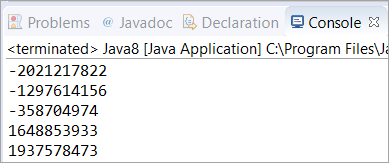Q #20) What is the difference between Intermediate and Terminal Operations in Stream?

Answer: All Stream operations are either Terminal or Intermediate. Intermediate Operations are the operations that return the Stream so that some other operations can be carried out on that Stream. Intermediate operations do not process the Stream at the call site, hence they are called lazy.

These types of operations (Intermediate Operations) process data when there is a Terminal operation carried out. Examples of Intermediate operation are map and filter.

Terminal Operations initiate Stream processing. During this call, the Stream undergoes all the Intermediate operations. Examples of Terminal Operation are sum, Collect, and forEach.

In this program, we are first trying to execute Intermediate operation without Terminal operation. As you can see the first block of code won’t execute because there is no Terminal operation supporting.

The second block successfully executed because of the Terminal operation sum().

 `import` `java.util.Arrays;``class` `Java8 {``    ``public` `static` `void` `main(String[] args) {``        ``System.out.println(``"Intermediate Operation won't execute"``);``        ``Arrays.stream(``new` `int``[] { ``0``, ``1` `}).map(i -> {``            ``System.out.println(i);``            ``return` `i;``            ``// No terminal operation so it won't execute``        ``});``        ``System.out.println(``"Terminal operation starts here"``);``        ``Arrays.stream(``new` `int``[] { ``0``, ``1` `}).map(i -> {``            ``System.out.println(i);``            ``return` `i;``            ``// This is followed by terminal operation sum()``        ``}).sum();``    ``}``}`

Output: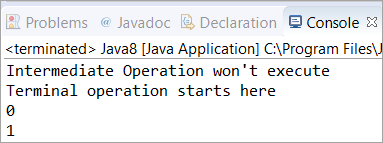Q #21) Write a Java 8 program to get the sum of all numbers present in a list?

Answer: In this program, we have used ArrayList to store the elements. Then, with the help of the sum() method, we have calculated the sum of all the elements present in the ArrayList. Then it is converted to Stream and added each element with the help of mapToInt() and sum() methods.

 `import` `java.util.*;``class` `Java8 {``    ``public` `static` `void` `main(String[] args) {``        ``ArrayList list = ``new` `ArrayList();``        ``list.add(``10``);``        ``list.add(``20``);``        ``list.add(``30``);``        ``list.add(``40``);``        ``list.add(``50``);``        ``// Added the numbers into Arraylist``        ``System.out.println(sum(list));``    ``}``    ``public` `static` `int` `sum(ArrayList list) {``        ``return` `list.stream().mapToInt(i -> i).sum();``        ``// Found the total using sum() method after ``        ``// converting it into Stream``    ``}``}`

Output: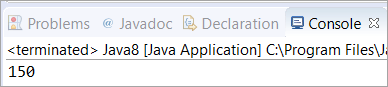Q #22) Write a Java 8 program to square the list of numbers and then filter out the numbers greater than 100 and then find the average of the remaining numbers?

Answer: In this program, we have taken an Array of Integers and stored them in a list. Then with the help of mapToInt(), we have squared the elements and filtered out the numbers greater than 100. Finally, the average of the remaining number (greater than 100) is calculated.

 `import` `java.util.Arrays;``import` `java.util.List;``import` `java.util.OptionalDouble;``public` `class` `Java8 {``    ``public` `static` `void` `main(String[] args) {``        ``Integer[] arr = ``new` `Integer[] { ``100``, ``100``, ``9``, ``8``, ``200` `};``        ``List list = Arrays.asList(arr);``        ``// Stored the array as list``        ``OptionalDouble avg = list.stream().mapToInt(n -> n * n).filter(n -> n > ``100``).average();``        ``        ``/* Converted it into Stream and filtered out the numbers``            ``which are greater than 100. Finally calculated the average ``        ``*/``        ``        ``if` `(avg.isPresent())``            ``System.out.println(avg.getAsDouble());``    ``}``}`

Output: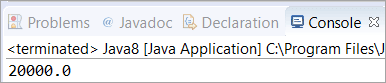Q #23) What is the difference between Stream’s findFirst() and findAny()?

Answer: As the name suggests, the findFirst() method is used to find the first element from the stream whereas the findAny() method is used to find any element from the stream.

The findFirst() is predestinarianism in nature whereas the findAny() is non-deterministic. In programming, Deterministic means the output is based on the input or initial state of the system.

Q #24) What is the difference between Iterator and Spliterator?

Answer: Below is the differences between Iterator and Spliterator.

IteratorSpliterator
It was introduced in Java version 1.2It was introduced in Java SE 8
It is used for Collection API.It is used for Stream API.
Some of the iterate methods are next() and hasNext() which are used to iterate elements.Spliterator method is tryAdvance().
We need to call the iterator() method on Collection Object.We need to call the spliterator() method on Stream Object.
Iterates only in sequential order.Iterates in Parallel and sequential order.

Q #25) What is the Consumer Functional Interface?

Answer: Consumer Functional Interface is also a single argument interface (like Predicate<T> and Function<T, R>). It comes under java.util.function.Consumer. This does not return any value.

In the below program, we have made use of the accept method to retrieve the value of the String object.

 `import` `java.util.function.Consumer;``public` `class` `Java8 {``    ``public` `static` `void` `main(String[] args) ``        ``Consumer str = str1 -> System.out.println(str1);``        ``str.accept(``"Saket"``);``        ``        ``/* We have used accept() method to get the ``         ``value of the String Object``         ``*/``    ``}``}`

Output: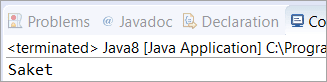Q #26) What is the Supplier Functional Interface?

Answer: Supplier Functional Interface does not accept input parameters. It comes under java.util.function.Supplier. This returns the value using the get method.

In the below program, we have made use of the get method to retrieve the value of the String object.

 `import` `java.util.function.Supplier;``public` `class` `Java8 {``    ``public` `static` `void` `main(String[] args) {``        ``Supplier str = () -> ``"Saket"``;``        ``System.out.println(str.get());``        ``        ``/* We have used get() method to retrieve the ``           ``value of String object str.``         ``*/``    ``}``}`

Output: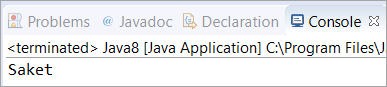Q #27) What is Nashorn in Java 8?

Answer: Nashorn in Java 8 is a Java-based engine for executing and evaluating JavaScript code.

Q #28) Write a Java 8 program to find the lowest and highest number of a Stream?

Answer: In this program, we have used min() and max() methods to get the highest and lowest number of a Stream. First of all, we have initialized a Stream that has Integers and with the help of the Comparator.comparing() method, we have compared the elements of the Stream.

When this method is incorporated with max() and min(), it will give you the highest and lowest numbers. It will also work when comparing the Strings.

 `import` `java.util.Comparator;``import` `java.util.stream.*;``public` `class` `Java8{``   ``public` `static` `void` `main(String args[]) {``        ``    ``Integer highest = Stream.of(``1``, ``2``, ``3``, ``77``, ``6``, ``5``)``                        ``.max(Comparator.comparing(Integer::valueOf))``                        ``.get();``    ``    ``/* We have used max() method with Comparator.comparing() method``       ``to compare and find the highest number ``    ``*/``         ``    ``Integer lowest = Stream.of(``1``, ``2``, ``3``, ``77``, ``6``, ``5``)``                        ``.min(Comparator.comparing(Integer::valueOf))``                        ``.get();``    ``    ``/* We have used max() method with Comparator.comparing() method``       ``to compare and find the highest number ``    ``*/``    ``    ``System.out.println(``"The highest number is: "` `+ highest);``    ``System.out.println(``"The lowest number is: "` `+ lowest);``   ``}``}`

Output: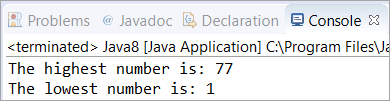Q #29) What is the Difference Between Map and flatMap Stream Operation?

Answer: Map Stream operation gives one output value per input value whereas flatMap Stream operation gives zero or more output value per input value.

Map Example – Map Stream operation is generally used for simple operation on Stream such as the one mentioned below.

In this program, we have changed the characters of “Names” into the upper case using map operation after storing them in a Stream and with the help of the forEach Terminal operation, we have printed each element.

 `import` `java.util.Arrays;``import` `java.util.List;``import` `java.util.stream.Collectors;``public` `class` `Map {``    ``public` `static` `void` `main(String[] str) {``        ``List Names = Arrays.asList(``"Saket"``, ``"Trevor"``, ``"Franklin"``, ``"Michael"``);``        ``List UpperCase = Names.stream().map(String::toUpperCase).collect(Collectors.toList());``        ``// Changed the characters into upper case after converting it into Stream``        ``UpperCase.forEach(System.out::println);``        ``// Printed using forEach Terminal Operation``    ``}``}`

Output: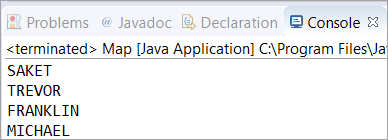flatMap Example – flatMap Stream operation is used for more complex Stream operation.

Here we have carried out flatMap operation on “List of List of type String”. We have given input names as list and then we have stored them in a Stream on which we have filtered out the names which start with ‘S’.

Finally, with the help of the forEach Terminal operation, we have printed each element.

 `import` `java.util.Arrays;``import` `java.util.List;``import` `java.util.stream.Collectors;``public` `class` `flatMap {``    ``public` `static` `void` `main(String[] str) {``        ``List> Names = Arrays.asList(Arrays.asList(``"Saket"``, ``"Trevor"``), Arrays.asList(``"John"``, ``"Michael"``),``                ``Arrays.asList(``"Shawn"``, ``"Franklin"``), Arrays.asList(``"Johnty"``, ``"Sean"``));``        ``        ``/* Created a “List of List of type String” i.e. List> ``           ``Stored names into the list``         ``*/``        ``List Start = Names.stream().flatMap(FirstName -> FirstName.stream()).filter(s -> s.startsWith(``"S"``))``                ``.collect(Collectors.toList());``        ``        ``/* Converted it into Stream and filtered ``            ``out the names which start with 'S'``         ``*/``        ``        ``Start.forEach(System.out::println);``        ``        ``/*``         ``Printed the Start using forEach operation``         ``*/``    ``}``}`

Output: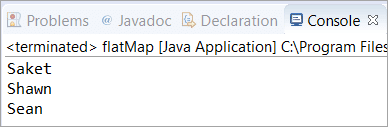Q #30) What is MetaSpace in Java 8?

Answer: In Java 8, a new feature was introduced to store classes. The area where all the classes that are stored in Java 8 are called MetaSpace. MetaSpace has replaced the PermGen.

Till Java 7, PermGen was used by Java Virtual Machine to store the classes. Since MetaSpace is dynamic as it can grow dynamically and it does not have any size limitation, Java 8 replaced PermGen with MetaSpace.

Q #31) What is the difference between Java 8 Internal and External Iteration?

Answer: The difference between Internal and External Iteration is enlisted below.

Internal IterationExternal Iteration
It was introduced in Java 8 (JDK-8).It was introduced and practiced in the previous version of Java (JDK-7, JDK-6 and so on).
It iterates internally on the aggregated objects such as Collection.It iterates externally on the aggregated objects.
It supports the Functional programming style.It supports the OOPS programming style.
Internal Iterator is passive.External Iterator is active.
It is less erroneous and requires less coding.It requires little more coding and it is more error-prone.

Q #32) What is JJS?

Answer: JJS is a command-line tool used to execute JavaScript code at the console. In Java 8, JJS is the new executable which is a JavaScript engine.

Q #33) What is ChronoUnits in Java 8?

Answer: ChronoUnits is the enum that is introduced to replace the Integer values that are used in the old API for representing the month, day, etc.

Q #34) Explain StringJoiner Class in Java 8? How can we achieve joining multiple Strings using StringJoiner Class?

Answer: In Java 8, a new class was introduced in the package java.util which was known as StringJoiner. Through this class, we can join multiple strings separated by delimiters along with providing prefix and suffix to them.

In the below program, we will learn about joining multiple Strings using StringJoiner Class. Here, we have “,” as the delimiter between two different strings. Then we have joined five different strings by adding them with the help of the add() method. Finally, printed the String Joiner.

In the next question #35, you will learn about adding prefix and suffix to the string.

 `import` `java.util.StringJoiner;``public` `class` `Java8 {``    ``public` `static` `void` `main(String[] args) {``        ``StringJoiner stj = ``new` `StringJoiner(``","``);``        ``// Separated the elements with a comma in between.``        ``        ``stj.add(``"Saket"``);``        ``stj.add(``"John"``);``        ``stj.add(``"Franklin"``);``        ``stj.add(``"Ricky"``);``        ``stj.add(``"Trevor"``);``        ``        ``// Added elements into StringJoiner “stj”``        ``        ``System.out.println(stj);``    ``}``}`

Output: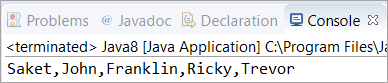Q #35) Write a Java 8 program to add prefix and suffix to the String?

Answer: In this program, we have “,” as the delimiter between two different strings. Also, we have given “(” and “)” brackets as prefix and suffix. Then five different strings are joined by adding them with the help of the add() method. Finally, printed the String Joiner.

 `import` `java.util.StringJoiner;``public` `class` `Java8 {``    ``public` `static` `void` `main(String[] args) {``        ``StringJoiner stj = ``new` `StringJoiner(``","``, ``"("``, ``")"``);``        ``        ``// Separated the elements with a comma in between.``        ``//Added a prefix "(" and a suffix ")"``        ``        ``stj.add(``"Saket"``);``        ``stj.add(``"John"``);``        ``stj.add(``"Franklin"``);``        ``stj.add(``"Ricky"``);``        ``stj.add(``"Trevor"``);``        ``        ``// Added elements into StringJoiner “stj”``        ``        ``System.out.println(stj);``    ``}``}`

Output: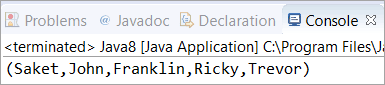Q #36) Write a Java 8 program to iterate a Stream using the forEach method?

Answer: In this program, we are iterating a Stream starting from “number = 2”, followed by the count variable incremented by “1” after each iteration.

Then, we are filtering the number whose remainder is not zero when divided by the number 2. Also, we have set the limit as ? 5 which means only 5 times it will iterate. Finally, we are printing each element using forEach.

 `import` `java.util.stream.*;  ``public` `class` `Java8 {  ``    ``    ``public` `static` `void` `main(String[] args){  ``        ``Stream.iterate(``2``, count->count+``1``) ``        ``        ``// Counter Started from 2, incremented by 1``        ``        ``.filter(number->number%``2``==``0``)  ``        ``        ``// Filtered out the numbers whose remainder is zero``        ``// when divided by 2``        ``        ``.limit(``5``)  ``        ``// Limit is set to 5, so only 5 numbers will be printed``        ``        ``.forEach(System.out::println);``    ``}  ``}`

Output: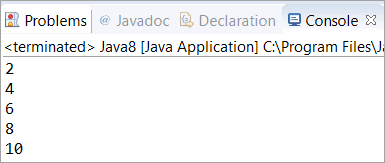Q #37) Write a Java 8 program to sort an array and then convert the sorted array into Stream?

Answer: In this program, we have used parallel sort to sort an array of Integers. Then converted the sorted array into Stream and with the help of forEach, we have printed each element of a Stream.

 `import` `java.util.Arrays;``public` `class` `Java8 {``    ``public` `static` `void` `main(String[] args) {``        ``int` `arr[] = { ``99``, ``55``, ``203``, ``99``, ``4``, ``91` `};``        ``Arrays.parallelSort(arr);``        ``// Sorted the Array using parallelSort()``        ``        ``Arrays.stream(arr).forEach(n -> System.out.print(n + ``" "``));``        ``/* Converted it into Stream and then``           ``printed using forEach */``    ``}``}`

Output: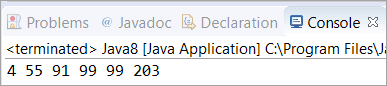Q #38) Write a Java 8 program to find the number of Strings in a list whose length is greater than 5?

Answer: In this program, four Strings are added in the list using add() method, and then with the help of Stream and Lambda expression, we have counted the strings who has a length greater than 5.

 `import` `java.util.ArrayList;``import` `java.util.List;``public` `class` `Java8 {``    ``public` `static` `void` `main(String[] args) {``        ``List list = ``new` `ArrayList();``        ``list.add(``"Saket"``);``        ``list.add(``"Saurav"``);``        ``list.add(``"Softwaretestinghelp"``);``        ``list.add(``"Steve"``);``        ``        ``// Added elements into the List``        ``long` `count = list.stream().filter(str -> str.length() > ``5``).count();``        ``        ``/* Converted the list into Stream and filtering out``           ``the Strings whose length more than 5``           ``and counted the length``           ``*/``        ``System.out.println(``"We have "` `+ count + ``" strings with length greater than 5"``);``    ``}``}`

Output: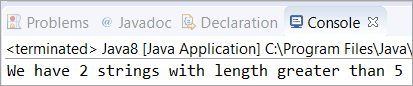Q #39) Write a Java 8 program to concatenate two Streams?

Answer: In this program, we have created two Streams from the two already created lists and then concatenated them using a concat() method in which two lists are passed as an argument. Finally, printed the elements of the concatenated stream.

 `import` `java.util.Arrays;``import` `java.util.List;``import` `java.util.stream.Stream;``public` `class` `Java8 {``    ``public` `static` `void` `main(String[] args) {``        ``List list1 = Arrays.asList(``"Java"``, ``"8"``);``        ``List list2 = Arrays.asList(``"explained"``, ``"through"``, ``"programs"``);``        ``Stream concatStream = Stream.concat(list1.stream(), list2.stream());``        ``        ``// Concatenated the list1 and list2 by converting them into Stream``        ``concatStream.forEach(str -> System.out.print(str + ``" "``));``        ``        ``// Printed the Concatenated Stream``        ``    ``}``}`

Output: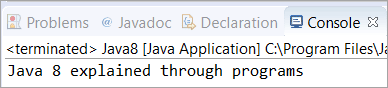Q #40) Write a Java 8 program to remove the duplicate elements from the list?

Answer: In this program, we have stored the elements into an array and converted them into a list. Thereafter, we have used stream and collected it to “Set” with the help of the “Collectors.toSet()” method.

 `import` `java.util.Arrays;``import` `java.util.List;``import` `java.util.Set;``import` `java.util.stream.Collectors;``public` `class` `Java8 {``    ``public` `static` `void` `main(String[] args) {``        ``Integer[] arr1 = ``new` `Integer[] { ``1``, ``9``, ``8``, ``7``, ``7``, ``8``, ``9` `};``        ``List listdup = Arrays.asList(arr1);``        ``        ``// Converted the Array of type Integer into List``        ``Set setNoDups = listdup.stream().collect(Collectors.toSet());``        ``        ``// Converted the List into Stream and collected it to “Set”``        ``// Set won't allow any duplicates``        ``        ``setNoDups.forEach((i) -> System.out.print(``" "` `+ i));``        ``        ``    ``}``}`

Output: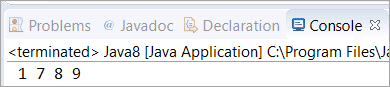### Conclusion

In this article, we have understood the new features introduced in Java 8. We have covered all major Java 8 interview questions and their answers in detail.

After perusing this instructional exercise, you probably acquired information about the new APIs for date-time control, new highlights of Java 8, new Streaming APIs alongside the able programming models according to the idea. These new ideas or the highlights are essential for the screening when you are up for the really difficult Java Positions.